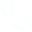# Print diamonds in JAVA (using for loops) with detailed comments

Print diamonds in JAVA (using for loops) with detailed notes

public static void main ( String[] args ) {
//diamond
int b = 1 ;
int d = 1 ;
//First, print the upper half of the rhombus, a triangle
for ( int i = 1 ; i < 8 ; i += 2 ) { //Specify that the maximum number of lines is less than 8, and the number of each line is the number of the previous line plus 2

for ( int n = 4 ; n > b; n--) { // output the number of spaces, the first line outputs 3, the second line outputs 2, and the third line outputs one
System.out.print ( " " );
}

for ( int y = 0 ; y < i ; y++) { // output the number of *, one in the first row, three in the second row, five in the third row, and seven in the fourth row
System.out.print ( "*" );
}

System.out.println ();
b += 1 ; // add 1 to b, so that the number of spaces output per line can be reduced

}
//Print the lower half of the rhombus, an inverted triangle
for ( int c = 1 ; c < 6 ; c += 2 ) {
for ( int y = 0 ; y < d; y++) { //The first line of the second half outputs one space, the second line outputs two spaces, and the third line outputs three spaces
System.out.print ( " " );
}
for ( int n = 6 ; n > c; n--) { //The first line of the second half outputs five *, the second line outputs three, and the last line outputs one
System.out.print ( "*" );
}
System.out.println ();
d += 1 ; // every time d+1, so that the number of spaces output per line can increase

}

}
}

## Related Articles

• ### A detailed explanation of Hadoop core architecture HDFS

Knowledge Base Team

• ### What Does IOT Mean

Knowledge Base Team

• ### 6 Optional Technologies for Data Storage

Knowledge Base Team

• ### What Is Blockchain Technology

Knowledge Base Team

## Explore More Special Offers

1. ### Short Message Service(SMS) & Mail Service

50,000 email package starts as low as USD 1.99, 120 short messages start at only USD 1.00Contact Us